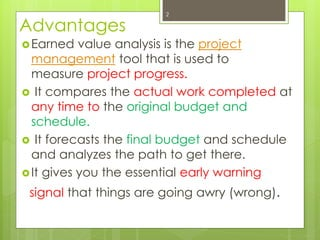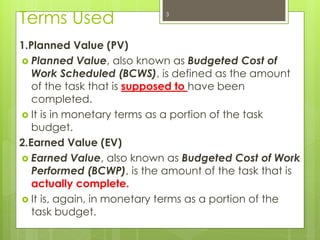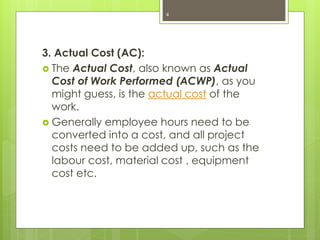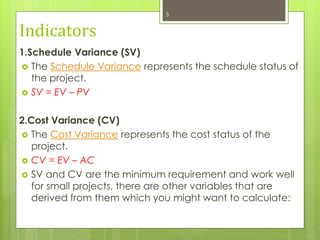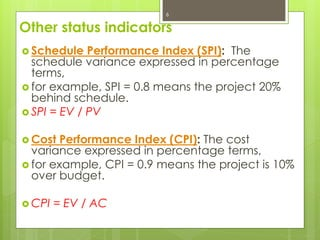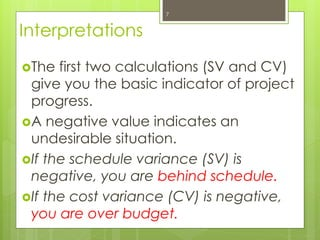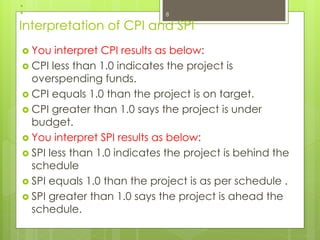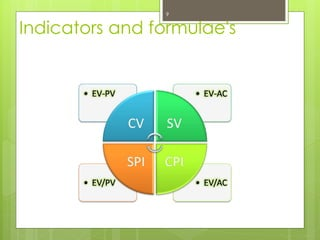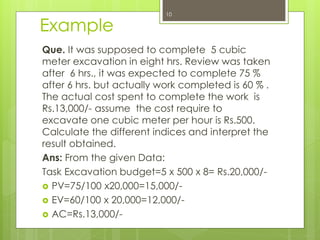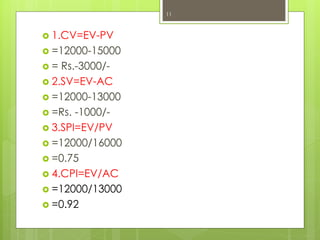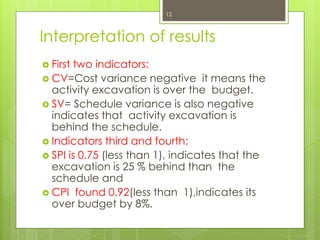1 de 12

### Earned value analysis

1. EARNED VALUE ANALYSIS BY Prof. Nagesh Suryawanshi
2. Advantages Earned value analysis is the project management tool that is used to measure project progress.  It compares the actual work completed at any time to the original budget and schedule.  It forecasts the final budget and schedule and analyzes the path to get there. It gives you the essential early warning signal that things are going awry (wrong). 2
3. Terms Used 1.Planned Value (PV)  Planned Value, also known as Budgeted Cost of Work Scheduled (BCWS), is defined as the amount of the task that is supposed to have been completed.  It is in monetary terms as a portion of the task budget. 2.Earned Value (EV)  Earned Value, also known as Budgeted Cost of Work Performed (BCWP), is the amount of the task that is actually complete.  It is, again, in monetary terms as a portion of the task budget. 3
4. 3. Actual Cost (AC):  The Actual Cost, also known as Actual Cost of Work Performed (ACWP), as you might guess, is the actual cost of the work.  Generally employee hours need to be converted into a cost, and all project costs need to be added up, such as the labour cost, material cost , equipment cost etc. 4
5. Indicators 1.Schedule Variance (SV)  The Schedule Variance represents the schedule status of the project.  SV = EV – PV 2.Cost Variance (CV)  The Cost Variance represents the cost status of the project.  CV = EV – AC  SV and CV are the minimum requirement and work well for small projects, there are other variables that are derived from them which you might want to calculate: 5
6. Other status indicators  Schedule Performance Index (SPI): The schedule variance expressed in percentage terms,  for example, SPI = 0.8 means the project 20% behind schedule.  SPI = EV / PV  Cost Performance Index (CPI): The cost variance expressed in percentage terms,  for example, CPI = 0.9 means the project is 10% over budget.  CPI = EV / AC 6
7. Interpretations The first two calculations (SV and CV) give you the basic indicator of project progress. A negative value indicates an undesirable situation. If the schedule variance (SV) is negative, you are behind schedule. If the cost variance (CV) is negative, you are over budget. 7
8. : Interpretation of CPI and SPI  You interpret CPI results as below:  CPI less than 1.0 indicates the project is overspending funds.  CPI equals 1.0 than the project is on target.  CPI greater than 1.0 says the project is under budget.  You interpret SPI results as below:  SPI less than 1.0 indicates the project is behind the schedule  SPI equals 1.0 than the project is as per schedule .  SPI greater than 1.0 says the project is ahead the schedule. 8
9. Indicators and formulae's 9
10. Que. It was supposed to complete 5 cubic meter excavation in eight hrs. Review was taken after 6 hrs., it was expected to complete 75 % after 6 hrs. but actually work completed is 60 % . The actual cost spent to complete the work is Rs.13,000/- assume the cost require to excavate one cubic meter per hour is Rs.500. Calculate the different indices and interpret the result obtained. Ans: From the given Data: Task Excavation budget=5 x 500 x 8= Rs.20,000/-  PV=75/100 x20,000=15,000/-  EV=60/100 x 20,000=12,000/-  AC=Rs.13,000/- Example 10
11.  1.CV=EV-PV  =12000-15000  = Rs.-3000/-  2.SV=EV-AC  =12000-13000  =Rs. -1000/-  3.SPI=EV/PV  =12000/16000  =0.75  4.CPI=EV/AC  =12000/13000  =0.92 11
12. Interpretation of results  First two indicators:  CV=Cost variance negative it means the activity excavation is over the budget.  SV= Schedule variance is also negative indicates that activity excavation is behind the schedule.  Indicators third and fourth:  SPI is 0.75 (less than 1), indicates that the excavation is 25 % behind than the schedule and  CPI found 0.92(less than 1),indicates its over budget by 8%. 12